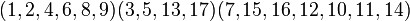# Order of permutation is lcm of cycle sizes

## Statement

### For a permutation on a finite set

Suppose$\sigma$ is a permutation on a finite set of size$n$ with Cycle type (?)$n = c_1 + c_2 + \dots + c_r$. Then, the order of$\sigma$ as an element of the symmetric group of degree$n$ is the lcm of$c_1,c_2,\dots,c_r$.

### For a permutation on an infinite set

Suppose$\sigma$ is a permutation on an infinite set with the property that every element is in a cycle of finite size. (Note that finitary permutations have this property, but infinitary permutations may also have this property). Then, if there is a uniform bound on the sizes of all the cycles, the element$\sigma$ has finite order and this order is the lcm of the sizes of all the cycles.

## Examples

### Illustrative examples

For instance, consider the permutation on the set$\{ 1,2,3,\dots,17 \}$ with cycle decomposition:$\! (1,2,4,6,8,9)(3,5,13,17)(7,15,16,12,10,11,14)$

The cycle type here is the unordered integer partition$17 = 7 + 6 + 4$. The order of the permutation is thus the lcm of$7,6,4$, which is 84.

Here's another example -- a permutation on$\{ 1,2,3, \dots, 17 \}$ with cycle decomposition:$\! (1,3,5,7)(2,4,8,6)(9,10,12)(11,13,15)(16,17)$

The cycle type here is$15 = 4 + 4 + 3 + 3 + 2 + 1$ (the point$\{ 14 \}$ is a fixed point). The order of the permutation is the lcm of$4,3,2,1$, which is 12.

Note from these examples that in case of repetitions in cycle sizes, we can just pick each element once when computing the lcm. Also, we can ignore the cycles of size 1.

### Comprehensive treatment of small degrees

In the right column links in the table below, you can see tabulated information on the orders of permutations by cycle type, along with how this order is computed as a lcm.

Degree Symmetric group Element structure page Section on conjugacy class structure interpreted as symmetric group
3 symmetric group:S3 element structure of symmetric group:S3 element structure of symmetric group:S3#Interpretation as symmetric group
4 symmetric group:S4 element structure of symmetric group:S4 element structure of symmetric group:S4#Interpretation as symmetric group
5 symmetric group:S5 element structure of symmetric group:S5 element structure of symmetric group:S5#Interpretation as symmetric group
6 symmetric group:S6 element structure of symmetric group:S6 element structure of symmetric group:S6#Interpretation as symmetric group
7 symmetric group:S7 element structure of symmetric group:S7 element structure of symmetric group:S7#Interpretation as symmetric group
8 symmetric group:S8 element structure of symmetric group:S8 element structure of symmetric group:S8#Interpretation as symmetric group